Home > AC > Chapter 11 > Lesson 11.1.3 > Problem11-35

11-35.
1. Solve for x. Use any method. Check your solutions by testing them in the original equation. Homework Help ✎

1.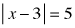2.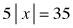3.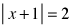4.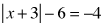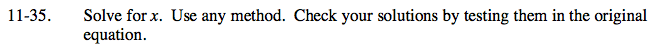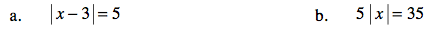x − 3 = 5 or x − 3 = −5
x = 8 or x = −2

Divide by 5, then solve.

x = ±7Use the same method as part (a).

x = 1 or −3

Can an absolute value equal a negative number?